Latest Banking jobs   »   Quantitative Aptitude Quiz For IBPS PO...

Quantitative Aptitude Quiz For IBPS PO Prelims 2021- 6th December

Directions (1–5): Given below in each question there are two statements (I) and (II). You must determine; which statement is enough to give the answer of question. Also, there are five alternatives given, you have to choose one alternative as your answer of the questions:

Q1. What is area of the rectangle.
I. Length is 50% more than breadth.
II. Perimeter of square is 48 cm and breadth of rectangle is equal to side of square.
(a) only statement I
(b) Only statement II
(c) Both I and II together
(d) Either I or II alone
(e) Both statements together are not sufficient

Q2. What is age of Rahul after 2 years.
I. Average age of Arun and Neeraj is 24 years and ratio of age of Rahul to Arun is 2 : 3.
II. Neeraj is 4 years elder than Satish and ratio of age of Satish to Rahul is 1 : 2
(a) only statement I
(b) Only statement II
(c) Both I and II together
(d) Both statements together are not sufficient
(e) Either I or II alone

Q3. What is the speed of boat in still water when the upstream speed of boat is equal to the speed of stream?
I. Time required to cover certain distance upstream is 24 sec.
II. Time required to cover certain distance downstream is 8 sec.
(a) only statement I
(b) Only statement II
(c) Both I and II together
(d) Both together are not sufficient
(e) Either I or II alone

Q4. Find out the length of train X given that speed of train X is 20 m/sec.
I. Train X crosses another train Y moving in opposite direction in 6 sec and the speed of train Y is 50% more than the speed of train X.
II. Length of train Y is 50% less than length of train X.
(a) Both I and II together
(b) Only statement I
(c) Only statement II
(d) Both I and II together are not sufficient
(e) Either I or II alone

Q5. What is the total strength of company Adda247.
I. Ratio of male to female employees are 1 : 2
II. Total females are 280 and males are 50% of female.
(a) Both I and II together
(b) Only statement I
(c) Only statement II
(d) Both I and II together are not sufficient
(e) Either I or II alone

Directions (6-10): In the given questions, two quantities are given, one as ‘Quantity I’ and another as ‘Quantity II’. You have to determine relationship between two quantities and choose the appropriate option:

Q6. Ratio of age of Anurag and Bhumi is 6 : 7 and Dharmendra : Ekta is 9 : 5. Ratio of average age of Anurag, Bhumi, Dharmendra& Ekta to age of Chiru is 9 : 10
Quantity I- If ratio of age of Bhumi and Dharmendra is 7 : 9 and age of Chiru is 6 years less than age of Dharmendra, then age of Chiru.
Quantity II- If ratio of age of Bhumi and Dharmendra is 7 : 9 and age of Chiru is 6 years less than age of Dharmendra, Average age of Anurag& Dharmendra.
(a) Quantity I > Quantity II
(b) Quantity I < Quantity II
(c) Quantity I ≥ Quantity II
(d) Quantity I ≤ Quantity II
(e) Quantity I = Quantity II or no relation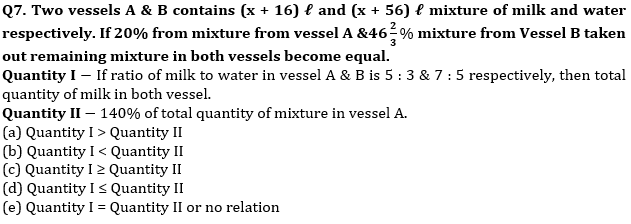Q8. Radius of cone is 4 cm less than side of square of which area is 324 cm2. Height of cone is 5 cm more than radius of circle, whose circumference is 44 cm.
Quantity I –Volume of cone.
Quantity II –2488 cm3
(a) Quantity I > Quantity II
(b) Quantity I < Quantity II
(c) Quantity I ≥ Quantity II
(d) Quantity I ≤ Quantity II
(e) Quantity I = Quantity II or no relation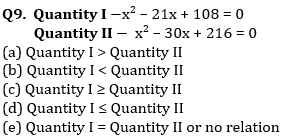Q10. 240 meters & 210 meters long trains running in opposite direction cross each other in 6 sec. Ratio between speed of longer train and smaller train is 7 : 8 and faster train cross a platform in 9 sec.
Quantity I – Time taken (in sec) by slower train to cross a bridge, which is 60 meters longer than platform.
Quantity II –16 sec
(a) Quantity I > Quantity II
(b) Quantity I < Quantity II
(c) Quantity I ≥ Quantity II
(d) Quantity I ≤ Quantity II
(e) Quantity I = Quantity II or no relation

Directions (11–15): In the given questions, two quantities are given one as ‘Quantity I’ and another as ‘Quantity II’. You have to determine relationship between two quantities and choose the appropriate option.

Q11.Quantity I: A train can cross a pole in 24 sec with a speed of 75 km/h. Length of train.
Quantity II: A train can cross a pole in 12 sec and a tunnel in 55.2 sec. If length of tunnel is 1800 m. length of train.
(a) Quantity I > Quantity II
(b) Quantity I < Quantity II
(c) Quantity I ≥ Quantity II
(d) Quantity I = Quantity II or no relation
(e) Quantity I≤ Quantity II

Q12. Quantity I: Marked price of article, if article is marked at 50% above cost price and on selling the article, profit earned is 20% and S. P is Rs 1020.
Quantity II: Total cost of fencing a square of side 37.5 meter and cost of wire is Rs 0.17 per centimeter.
(a) Quantity I > Quantity II
(b) Quantity I < Quantity II
(c) Quantity I ≥ Quantity II
(d) Quantity I = Quantity II or no relation
(e) Quantity I≤ Quantity II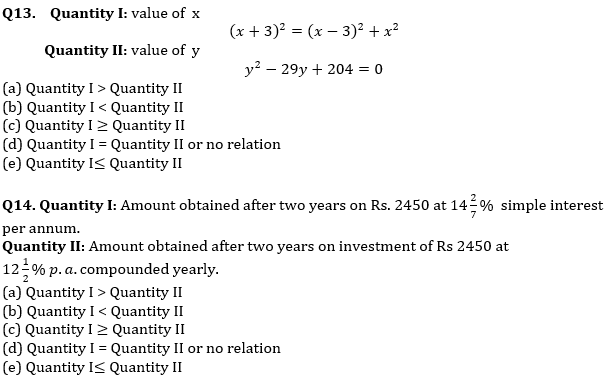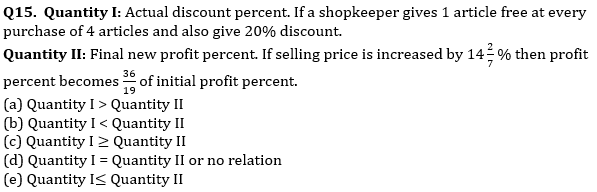Solutions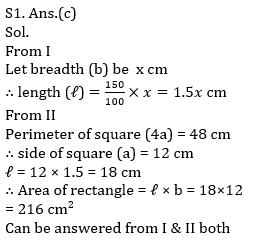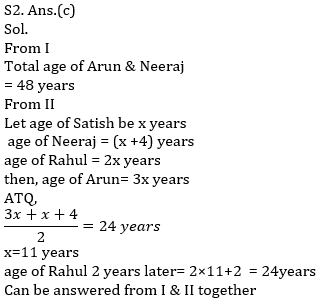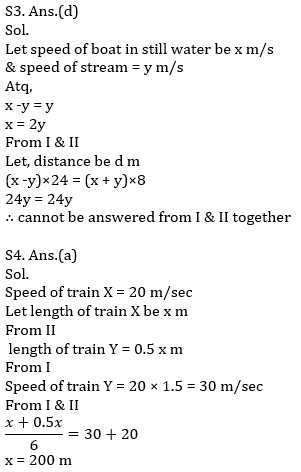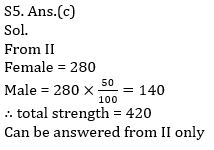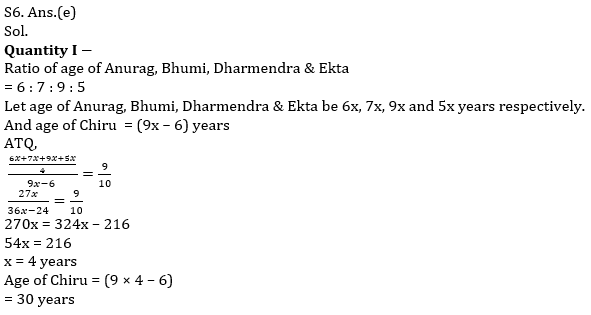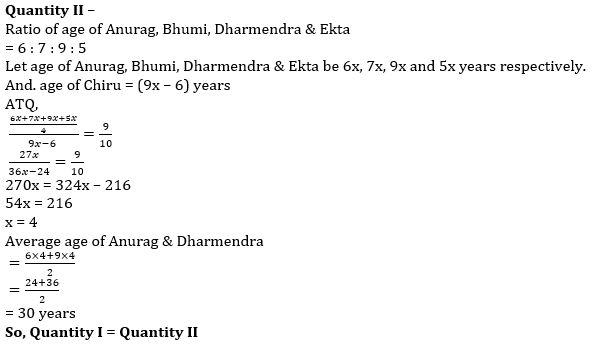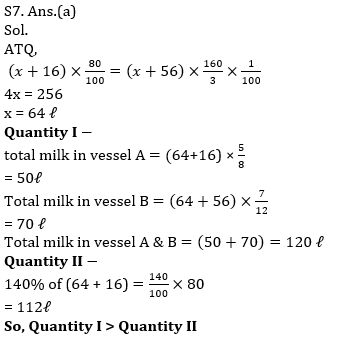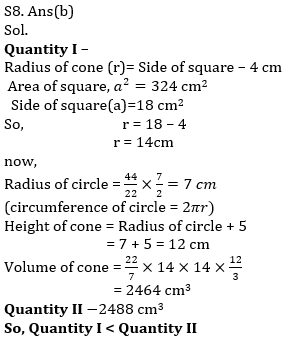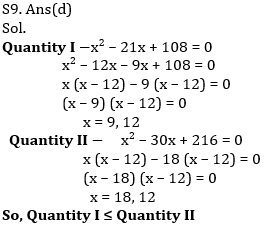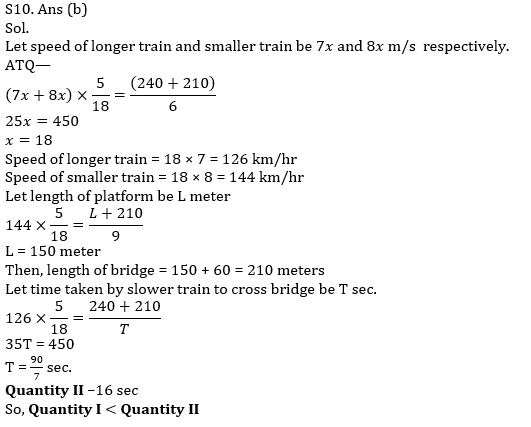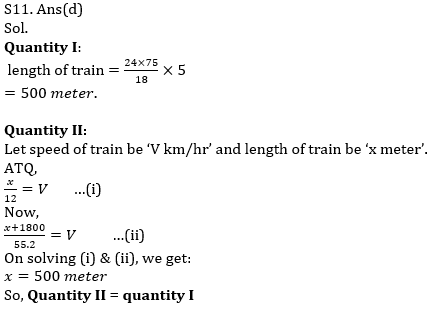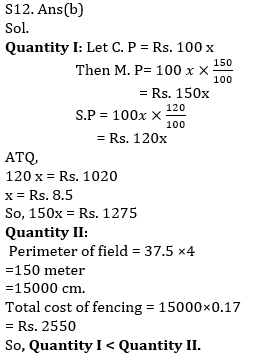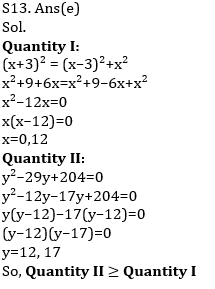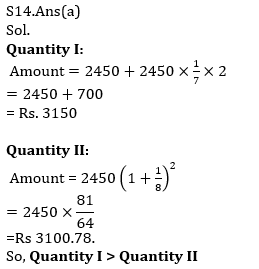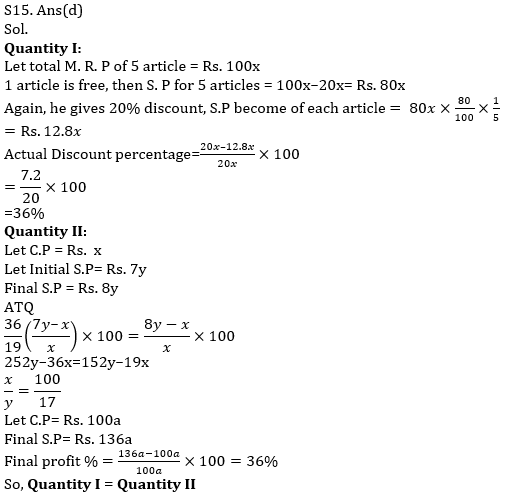×

Thank You, Your details have been submitted we will get back to you.Join India's largest learning destination

What You Will get ?

•Job Alerts
•Daily Quizzes
•Subject-Wise Quizzes
•Current Affairs
•Previous year question papers
•Doubt Solving session

ORJoin India's largest learning destination

What You Will get ?

•Job Alerts
•Daily Quizzes
•Subject-Wise Quizzes
•Current Affairs
•Previous year question papers
•Doubt Solving session

ORJoin India's largest learning destination

What You Will get ?

•Job Alerts
•Daily Quizzes
•Subject-Wise Quizzes
•Current Affairs
•Previous year question papers
•Doubt Solving session

Enter the email address associated with your account, and we'll email you an OTP to verify it's you.Join India's largest learning destination

What You Will get ?

•Job Alerts
•Daily Quizzes
•Subject-Wise Quizzes
•Current Affairs
•Previous year question papers
•Doubt Solving session

Enter OTP

Please enter the OTP sent to
/6

Did not recive OTP?

Resend in 60sJoin India's largest learning destination

What You Will get ?

•Job Alerts
•Daily Quizzes
•Subject-Wise Quizzes
•Current Affairs
•Previous year question papers
•Doubt Solving sessionJoin India's largest learning destination

What You Will get ?

•Job Alerts
•Daily Quizzes
•Subject-Wise Quizzes
•Current Affairs
•Previous year question papers
•Doubt Solving session

Almost there

+91Join India's largest learning destination

What You Will get ?

•Job Alerts
•Daily Quizzes
•Subject-Wise Quizzes
•Current Affairs
•Previous year question papers
•Doubt Solving session

Enter OTP

Please enter the OTP sent to Edit Number

Did not recive OTP?

Resend 60

By skipping this step you will not recieve any free content avalaible on adda247, also you will miss onto notification and job alerts

Are you sure you want to skip this step?

By skipping this step you will not recieve any free content avalaible on adda247, also you will miss onto notification and job alerts

Are you sure you want to skip this step?